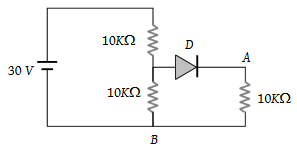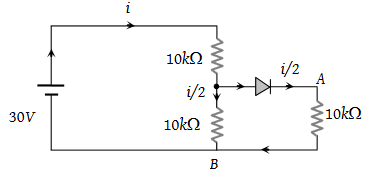In the given figure, potential difference between A and B is(1) 0

(2) 5 volt

(3) 10 volt

(4) 15 volt

(3)Equivalent resistance $R=10+\frac{10}{2}=15\text{\hspace{0.17em}}k\Omega$

Current i = $\frac{30}{15}=2×{10}^{-3}A$

Hence, potential difference between A and B

$V=\left(\frac{2×{10}^{-3}}{2}\right)×10×{10}^{3}=10\text{\hspace{0.17em}}Volt\text{.}$

Difficulty Level:

• 24%
• 19%
• 47%
• 12%